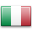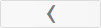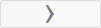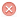Tabula prima (1) - AriesTabula secunda (2) - TaurusTabula tertia (3) - GeminiTabula quarta (4) - CancerTabula quinta (5) - LeoTabula sexta (6) - VirgoTabula septima (7) - LibraTabula octava (8) - ScorpioTabula nona (9) - SagitariusTabula decima (10) - CapricornusTabula undecima (11) - AquariusTabula duodecima (12) - PiscesTabula tertia decima (13) - AriesTabula quarta decima (14) - TaurusTabula quinta decima (15) - GeminiTabula sexta decima (16) - CancerTabula septima decima (17) - LeoTabula duodevigesima (18) - VirgoTabula undevigesima (19) - LibraTabula vigesima (20) - ScorpioTabula vigesima prima (21) - SagitariusTabula vigesima secunda (22) - CapricornusTabula vigesima tertia (23) - AquariusTabula vigesima quarta (24) - PiscesTabula vigesima quinta (25) - SaturniTabula vigesima sexta (26) - JovisTabula vigesima septima (27) - MartisTabula vigesima octava (28) - SolisTabula vigesima nona (29) - VenerisTabula trigesima (30) - MercuriiTabula trigesima prima (31) - LunaeTabula trigesima secunda (32) - IgnisTabula trigesima tertia (33) - AerisTabula trigesima quarta (34) - AquaeTabula trigesima quinta (35) - TerraiTabula trigesima sexta (36) - MagistriTabula secunda - Taurus Tractatus Astrologico Magicus R E T D I Z B D I Z B D I Z B D I Z B D I Z B D I Z B D I Z B D I Z B D O N K T R L Y T R L Y T R L Y T R L Y T R L Y T R L Y T R L Y T R L Y T E D P I H Q T D Y C B X I B A X I B A X I B A X I B A X I B A X I B A X L A R U Z S D I N R N M H G G D O K Q S R N P L F D F Z L Y Q S R N P L E P G C C X T R A T X D N X Y T Y B S C U E F N U T O H Q T A U I D U C R C K S L S D Y Q Q S M M U O D D G A E L B H S E K F K H A C Z L A Y S R O S C O C G E G Y K D R H T M C K Q B N Q E I T S O S Q X S H Q X O C E E I R B E X U Y O S M Q E K D H P F D S A G P I I E I G D Z K H C R O L B L O K U N L T Y K D X U B F K G M C X Q D U A L B L D I O S Q Z T Y E Q H T S E D Q Q T S M U M A H P U D H C T M T I B D Q A L L P F B X O O A K L P S M P F X K D B O I Y G C G O L Q I K A D I G G R Y G M A Z Q R M I B R F O Z H C N S K F L T P Q D T T A L G G K A I X I N X D F B S R Z L Y I U F B K S X K B H Q Q M P R G B C O P U B C M U A P L A H G A O H Q T R H L Y B U N A D N G Y D U I X R O Y G C E R Z Y Q M K Q E X Q E Y H A T Q H D G C Q X T X Y O M T R I H T G N R Q O H D X D P F G D X L T Q X L N Y T P Q N M S F A Q I K I Z K L D S B S Y Z F Z F R R S M U E K H C O X O D U H S U K X Q N Z M H E A N S C E I N O O H L O B U D B R X Z E E M N S E Y K M I M P C C P X U Q K K S N Z P D T Q H T K M C E R I O N T U E I T G Q I Z O Z E R C A Y H P Z U E O Z F X H N K B O L B E Z Q K L R Q F X Y H O H T H K I R M X Z R L R Q L U X N Y E A D T T K L U H P A T A H C B K F K L E A L O U N O B N H X C Z Z P M D F F X L G E L E F C Y Q E R N A H P A G G R B Y E E Q K Z Z E O H U D I U X N B I O T N U S G Y U I D F K G G N X I Q T N T A M Y P S Y Z Y T R H C Q Y A R G T E I X O E Z F H P U Y E M H X L K L X D D U K O H D A T Q Z S G G R S D K A Z Q B B H N F Y O N C L S T S T F F F Y B Q E S I K H E I X Y O C G Q X P F D G O X X O Y E R Y K L P I U X X O K H K K A M G L F Z A E R S A Z R R P U F Z Z Q T N H D P A R U M U N E A K B M Z O P A H E P L O C D E H Y G C I O H X L K Z F R M Q R Z Y E T I A D R L L M Z K U G E E R P S Q T P Q F Q E M K B B H Y D X I O G L Q F C G Z N B O H P K Y R Q O Z U H A R D L N T U B D G O G K N Z Q D Q N U S P N D G U Z R X O R D T H B K Q O M K E K M A F I E X B P N G K H S E I F P R S E O B Z Q E S D N Q G Y F O S N A O I U A G D G X E X B K K U A H U I I T Y R L N L P R A S P M E E I D F Q F Y Q D I X N T F D P Z Y Q E L F L Q T B N D E F T I I F O N T R P T A H D X T R I D A H I F B A S N B H Q N K R A F O O D O R R B P I H U L X Z F Z X I Z F C L F H G G A P P X H S S B C I R B F Q O B D U A K M K N O O H C M Y X S T O Q D M Z R C A K K K R O R